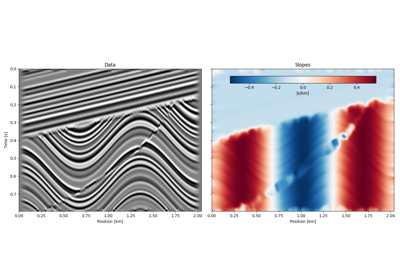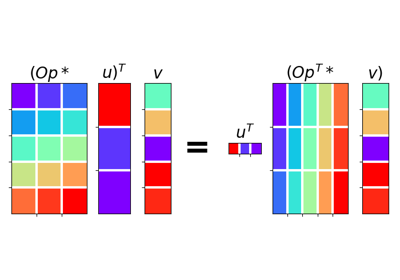# pylops.utils.dottest#

pylops.utils.dottest(Op, nr=None, nc=None, rtol=1e-06, atol=1e-21, complexflag=0, raiseerror=True, verb=False, backend='numpy')[source]#

Dot test.

Generate random vectors $$\mathbf{u}$$ and $$\mathbf{v}$$ and perform dot-test to verify the validity of forward and adjoint operators. This test can help to detect errors in the operator implementation.

Parameters
Oppylops.LinearOperator

Linear operator to test.

nrint

Number of rows of operator (i.e., elements in data)

ncint

Number of columns of operator (i.e., elements in model)

rtolfloat, optional

Relative dottest tolerance

atolfloat, optional

Absolute dottest tolerance .. versionadded:: 2.0.0

complexflagbool, optional

Generate random vectors with

• 0: Real entries for model and data

• 1: Complex entries for model and real entries for data

• 2: Real entries for model and complex entries for data

• 3: Complex entries for model and data

raiseerrorbool, optional

Raise error or simply return False when dottest fails

verbbool, optional

Verbosity

backendstr, optional

Backend used for dot test computations (numpy or cupy). This parameter will be used to choose how to create the random vectors.

Returns
passedbool

Passed flag.

Raises
AssertionError

If dot-test is not verified within chosen tolerances.

Notes

A dot-test is mathematical tool used in the development of numerical linear operators.

More specifically, a correct implementation of forward and adjoint for a linear operator should verify the following equality within a numerical tolerance:

$(\mathbf{Op}\,\mathbf{u})^H\mathbf{v} = \mathbf{u}^H(\mathbf{Op}^H\mathbf{v})$

## Examples using pylops.utils.dottest#Normal Moveout (NMO) Correction

Normal Moveout (NMO) CorrectionSeislet transform

Seislet transform02. The Dot-Test

02. The Dot-Test